# Zeroth-Order Solution

To zeroth order in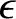, Equation (11.72) yields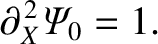(11.100)

Solving this equation subject to the boundary condition (11.83), we obtain(11.101)

Thus, we again conclude that, to lowest order in our expansion, the magnetic flux-surfaces in the island region have the constant-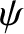structure pictured in Figure 5.7. The island O-points correspond to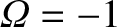and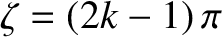(where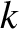is an integer), the X-points correspond to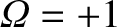and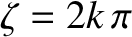, and the magnetic separatrix corresponds to.

To zeroth order in, Equation (11.71) yields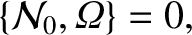(11.102)

where use has been made of Equation (11.101). Given that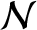is an odd function of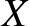, it follows that(11.103)

By symmetry,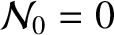inside the magnetic separatrix of the island chain. Let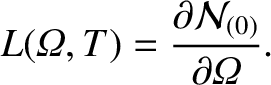(11.104)

Note that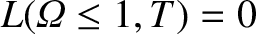. Equations (11.84) and (11.101) imply that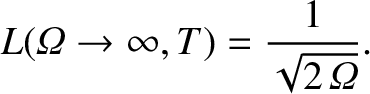(11.105)

To zeroth order in, Equation (11.68) yields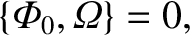(11.106)

where use has been made of Equations (11.101) and (11.103). Given that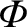is an odd function of, it follows that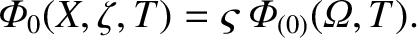(11.107)

By symmetry,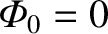inside the magnetic separatrix of the island chain. Let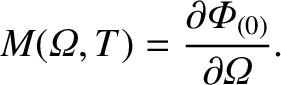(11.108)

Note that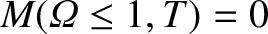. Equations (11.85) and (11.101) imply that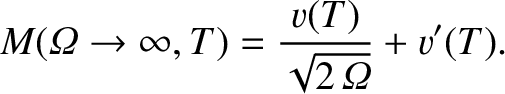(11.109)

To zeroth order in, Equation (11.69) yields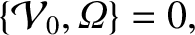(11.110)

where use has been made of Equations (11.101), (11.103), and (11.107). Given that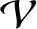is an even function of, we can write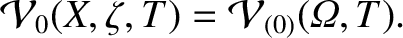(11.111)

Finally, to zeroth order in, Equation (11.70) gives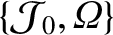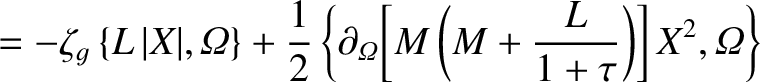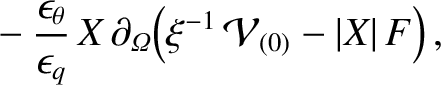(11.112)

where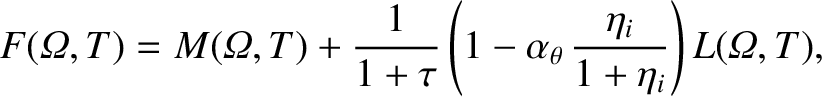(11.113)

and use has been made of Equations (11.101), (11.103), (11.104), (11.107), and (11.108). Moreover,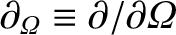. Note that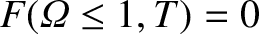.

Let us write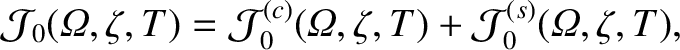(11.114)

where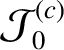has the symmetry of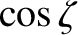, whereas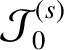has the symmetry of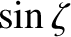. It follows from Equations (11.112) and (11.114) that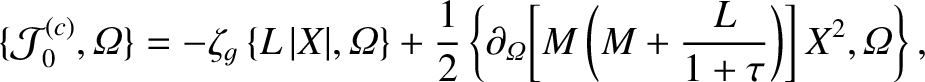(11.115)

which implies that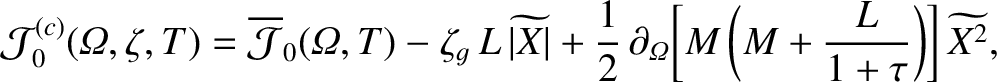(11.116)

where theoperator is defined in Section 8.6, and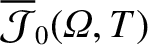is an undetermined flux-surface function.

Equations (11.112) and (11.114) also yield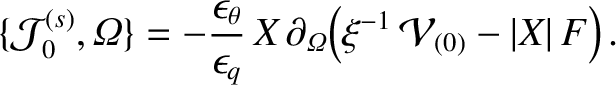(11.117)

The flux-surface average (see Section 8.6) of the previous equation gives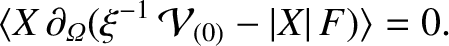(11.118)

Inside the separatrix of the magnetic island chain, recalling that, the previous equation reduces to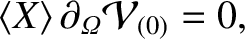(11.119)

whereas outside the separatrix, it gives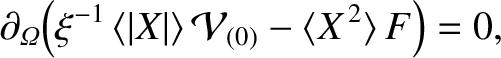(11.120)

where use has been made of the easily proved identity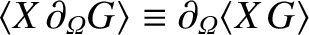. Thus, we conclude that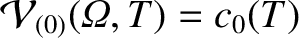(11.121)

inside the separatrix, and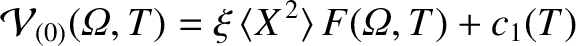(11.122)

outside the separatrix. Here, use has been made of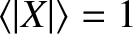outside the separatrix. However, the boundary condition (11.86), combined with Equations (11.101), (11.105), (11.109), and (11.113), implies that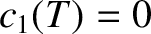. Finally, Equations (11.117), (11.121), and (11.122) yield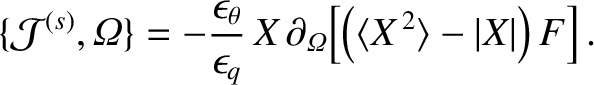(11.123)